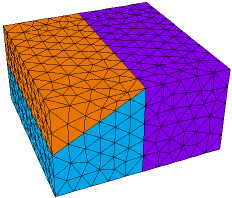﻿ Sample Problems > Usage > 3D_domains > 3d_pinchout

# 3d_pinchout

Navigation:  Sample Problems > Usage > 3D_domains >

# 3d_pinchout{ 3D_PINCHOUT.PDE    This problem demonstrates the merging  of extrusion surfaces and the  'Pinch-Out' of a layer. }     title '3D Layer Pinch-out Test'   coordinates    cartesian3   variables    Tp   select    ngrid=5 { reduce mesh size for example }   definitions    long = 1    wide = 1K = 1     { thermal conductivity default -- other values supplied later: }

Q = 10*exp(-x^2-y^2-z^2) { thermal source }

z1 = 0

z2       { surface will be defined later in each region: }

z3 = 1

initial values

Tp = 0.

equations

Tp:  div(k*grad(Tp)) + Q = 0       { the heat equation }

extrusion z = z1,z2,z3     { divide Z into two layers }

boundaries

surface 1 value(Tp)=0       { fix bottom surface temp }

surface 3 value(Tp)=0       { fix top surface temp }

Region 1           { define full domain boundary in base plane }

z2 = 1       { surface 2 merges with surface 3 in this region }

start(-wide,-wide)

value(Tp) = 0         { fix all side temps }

line to (wide,-wide)   { walk outer boundary in base plane }

to (wide,wide)

to (-wide,wide)

to close

Region 2           { Overlay a second region in left half.

This region delimits the area in which surfaces 2 and 3 differ.

Surfaces meet at the region boundary.  }

z2 = 1 + x/2

layer 2 k=0.1   { redefine conductivity in layer 2 of region 2 }

start(-wide,-wide)

line to (0,-wide)             { walk left half boundary in base plane }

to (0,wide)

to (-wide,wide)

to close

monitors

grid(x,z) on y=0

plots

grid(x,z) on y=0

contour(Tp) on y=0 as "ZX Temp"

end